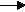gcsescience.com                                          27                                          gcsescience.com

Acids and Alkalis

Neutralisation.

An acid plus an alkali makes a salt plus water.

Hydrochloric acid makes chlorides.
Sulfuric acid makes sulfates. Nitric acid makes nitrates.

This is the balanced chemical equation for the reaction of
hydrochloric acid and sodium hydroxide.

hydrochloric acid  sodium hydroxidesodium chloride  +  water.
HCl(aq)          +           NaOH(aq)NaCl(aq)      +  H2O(l)

This is the balanced chemical equation for the reaction of
sulfuric acid and potassium hydroxide.

sulfuric acid potassium hydroxidepotassium sulfate  +  water.
H2SO4(aq)    +              2KOH(aq)K2SO4(aq)        +  2H2O(l)

When
ammonia is used as the alkali,
ammonium hydroxide is formed in solution.

This is the balanced chemical equation for the reaction of
sulfuric acid and ammonium hydroxide.

sulfuric acid  +  ammonium hydroxideammonium sulfate  +  water.
H2SO4(aq)    +       2
NH4OH(aq)(NH4)2SO4(aq)    +  2H2O(l)

The salt formed here is ammonium sulfate.
Ammonium salts are used as fertilisers.

All of the salts made above are soluble.
Pure samples of the salt can be made by using a titration.

gcsescience.com    The Periodic Table    Index    Neutralisation Quiz    gcsescience.com

Copyright © 2015 gcsescience.com. All Rights Reserved.